Courses

# Proving Techniques Computer Science Engineering (CSE) Notes | EduRev

## Computer Science Engineering (CSE) : Proving Techniques Computer Science Engineering (CSE) Notes | EduRev

The document Proving Techniques Computer Science Engineering (CSE) Notes | EduRev is a part of the Computer Science Engineering (CSE) Course Theory of Computation.
All you need of Computer Science Engineering (CSE) at this link: Computer Science Engineering (CSE)

Part II. Proving Techniques
An important requirement for learning this subject is the ability to follow proofs. There are three fundamental techniques
to proof. They are,
1. Principle of mathematical induction,
2. Pigeonhole principle,
3. Diagonalization principle.

2 Principle of Mathematical Induction
Let we want to show that property P holds for all natural numbers. To prove this property, P using mathematical induction,
following are the steps:
Basic Step:
First show that property P is true for 0 or 1.
Induction Hypotheis:
Assume that property P holds for n.
Induction step:
Using induction hypothesis, show that P is true for n+1.
Then by the principle of mathematical induction, P is true for all natural numbers.

Examples
Example 1:
Prove using mathematical induction, n− 4nis divisible by 3 for n>=0.
Basic step:
For n=0,
n4 − 4n2 =0, which is divisible by 3.
Induction hypothesis:
Let n4 − 4nis divisible by 3.
Induction step:
(n + 1)4 − 4(n + 1)2
= [(n + 1)2]2 − 4(n + 1)2
= (n2 + 2n + 1)− (2n + 2)2
= (n2 + 2n + 1 + 2n + 2)(n2 + 2n + 1 − 2n − 2)
= (n+ 4n + 3)(n2 − 1)
= n4 + 4n3 + 3n2 − 3 − 4n − n2
= n4 + 4n3 + 2n2 − 4n − 3
= n4 + 4n− 4n2 + 6n2 − 4n − 3
= n−4n2 + 6n2− 3 + 4n3−4n
= (n2−4n2) + (6n2)− (3) + 4(n3−n)
(n2−4n2) is divisible by 3 from our hypothesis.
6n2,3 are divisible by 3.

We need to prove that 4(n3−n) is divisible by 3.
Again use mathematical induction.
Basic step:
For n = 0,
4(0-0) = 0 is divisible by 3.
Induction hypothesis:
Let 4(n3−n) is divisible by 3.
Induction step:
4[(n + 1)3−(n + 1)]
= 4[(n3 + 3n2 + 3n + 1)−(n + 1)]
= 4[n3 + 3n2 + 3n + 1−n− 1]
= 4[n3 + 3n2 + 2n]
= 4[n3−n + 3n2 + 3n]
= 4(n3−n) + 4.3n2 + 4.3n4(n3−n) is divisible by 3 from our hypothesis.
4.3n2is divisible by 3.4.3n is divisible by 3.Thus we can say that
= (n2−4n2) + (6n2)− (3) + 4(n3−n) is divisible by 3.
That is,
n4 − 4nis divisible by 3.

Example 2:
Prove using mathematical induction:
1 + 2 + 3 + .......+n=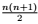LetP(n) = 1 + 2 + 3 + .......+n=Basic Step:
For n=1,
LHS = 1
RHS = 1(1+1)/2=1
Induction hypothesis;
Assume that P(n) is true for n=k,
Then,
1 + 2 + 3 + .......+k=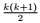Induction step:
Show that P(n) is true for n=k+1.
1 + 2 + 3 + ....... + k + (k + 1)
=+ k + 1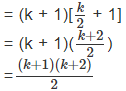That is, P(n) is true for n=k+1.
Thus by using the principle of mathematical induction, we proved
1 + 2 + 3 + ....... + n =Example 3:
Prove using the principle of mathematical induction,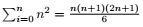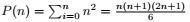Basic step:
For n=1,
LHS = 12 = 1
RHS=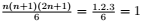P(n) is true for n=1.
Induction hypothesis:
Assume that result is true for n=k.
That is,
12 + 2+ ...... + k2 =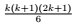Induction step:
Prove that result is true for n=k+1.
12 + 22 + ...... + k2 + (k + 1)2 =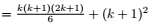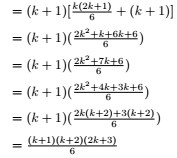Thus it is proved.

Exercise:
1. Prove the following by principle of induction: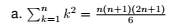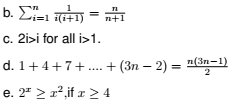f. 102n − 1 is divisible by 11 for all n>1
g. 2n > n, for all n>1.

3 Diagonalization Principle
Let s be a non empty set and R any relation on S.
Let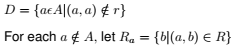Then diagonalization principle states that D is different from each Ra.
OR
Diagonalization principle states that the complement of the diagonal is different from each row.
For example,
Let S = {a, b, c, d}
R = { (a,a), (b,c), (b,d), (c,a), (c,c), (c,d), (d,a), (d,b) }
The above relation R is shown in matrix from as follows: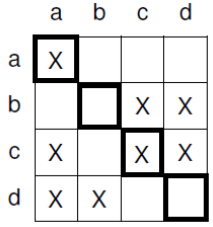Diagonal elements are marked.
From the figure, Ra = {a}
Rb = {c, d}
Rc = {a, c, d}
Rd = {a, b}
Complement of the diagonal is,
D = {b, d}
That is,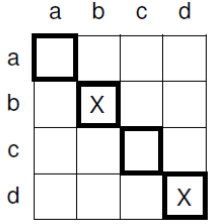If we compare each of the above Ra, Rb, Rc, Rd with D, we can see that D is different from each Ra. Thus complement
of the diagonal is distinct from each row.

Note:
Following information is needed to solve the example given below:
9’s complement of a number
9’s complement of 276 is 723.
9’s complement of 425 is 574.
9’s complement of 793 is 206.

Example 1:
Prove that the set of real numbers between 0 and 1 is uncountable.
An example for a real number between 0 and 1 is .34276
Let we represent a real number between 0 and 1 as
x = .x0x1x2x3..........
where each xi is a decimal digit.

Let f(k) be an arbitrary function from natural numbers to the set [0,1].
We can arrange the elements in a 2d array as,
f(0): . x00 x01 x02 x03 _ _ _
f(1): . x10 x11 x12 x13 _ _ _
f(2): . x20 x21 x22 x23 _ _ _
_
_
f(n): . xn0 xn1 xn2 xn3 _ _ _
where xni is the ith digit in the decimal expansion of f(n).
Next we find the complement of the diagonal (9’s complement) as follows:
Y = .y0y1y2.........
where yi =9’s complement of xii
[Find out the 9’s complement of x00, x11, x22, x33......xnn]

From the diagonalisation principle, it is clear that the complement of the diagonal is different from each row.
Here it is clear that Y is different from each f(i) in at least one digit. Y ∉  f(i). Hence Y cannot be present in the above
array.
This means that the set of real numbers between 0 and 1 is countably infinite or not countable.

For instance suppose we arrange the real numbers as,
f(0): . 9 4 2 4 _ _ _
f(1): . 6 3 6 2 _ _ _
f(2): . 2 8 6 4 _ _ _
f(3) . 6 5 3 2 _ _ _
_ . _ _ _ _ _ _ _
f(n): . 4 1 5 7 _ _ _
Here the diagonal is 9 3 6 2.......
The complement (9’s complement) of the diagonal is 0 6 3 7........
The real number .0637... is not in the above table.
Thus It is clear that this value is distinct from each row, f(0), f(1), f(2)...f(n).

4 Pigeonhole PrincipleA pigeonhole is a hole for pigeons to nest.
From the above diagram, it is clear that if 10 pigeons are put into 9 pigeonholes, then one pigeonhole must contain more than one item. The  pigeonhole principle states that if n pigeons are put into m pigeonholes with n>m, then at least one pigeonhole must contain more than one pigeon.  Thus if S1 and S2 are two non empty finite sets and |S1| > |S2|, then there is no one-to-one function from S1 to S2. Pigeonhole principle can be  used to show that certain languages are not regular. We will use pigeonhole principle in
the topic pumping lemma later.

Offer running on EduRev: Apply code STAYHOME200 to get INR 200 off on our premium plan EduRev Infinity!

## Theory of Computation

18 videos|43 docs|39 tests

,

,

,

,

,

,

,

,

,

,

,

,

,

,

,

,

,

,

,

,

,

;